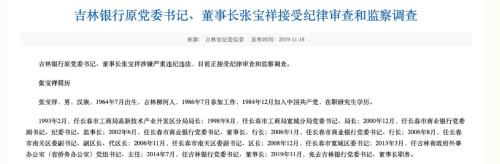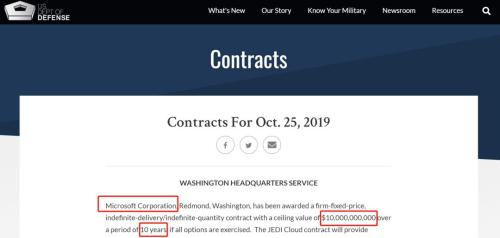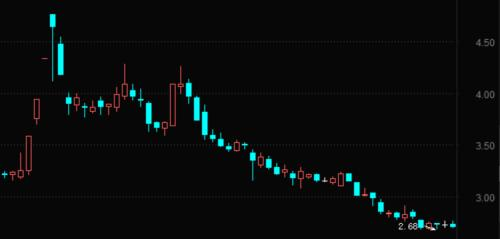## 头条今年稳外资目标能够实现

“虽然全球跨境投资整体不景气，但中国吸收外资额不仅没下降，反而稳中有升。目前全球投资者对华投资仍保持信心，预计今年稳外资目标能够实现。”11月18日，商务部就利用外资工作情况举办专题发布会，商务部外资司司长宗长青会上介绍。今年1-10月，我国实际使用外资（FDI）总体保持平稳增长，同比增长6.6%。进入11月，随着20条稳外资新政、营商环境优化举措相继发布，加之第二届进博会成功举办的放大和外溢效应，中国市场对外资的吸引力进一步加强。明年起，外商投资法将全面施行，释放更大改革红利。商务部外资司副司长叶威对此表示，目前将开展三方面的工作保障法规实施：一是制定配套条例，相关司法解释也在抓紧制定；二是清理相关规定；三是开展培训解读。10月FDI增长7.4%在刚落幕不久的第二届进博会上，来自美国的全球放疗领域龙头企业——瓦里安医疗全球政府事务高级副总裁魏安卓透露，今年他们将中国生产基地的产能扩大了30%，并计划在2020年将固定资产投资增加3倍，扩建生产线和瓦里安研发中心。无独有偶，跨国药企阿斯利康也在进博会上与上海市政府达成战略合作，将现有阿斯利康上海研发平台升级为全球研发中心，并成立AI（人工智能）创新中心，正式落户上海市静安区。在11月18日的发布会上，宗长青强调，我国连续两届成功举办了中国国际进口博览会，对于引进外资产生了明显的溢出效应。参展的外国企业将进博会作为深入了解中国市场、寻找在华投资机遇的重要平台。例如，首届进博会后，包括苹果、迪卡侬在内的多家世界500强和行业龙头企业，在中国设立了地区总部或研发中心。进博会的火爆程度反映出了外企对中国市场的青睐。商务部11月18日公布的FDI数据显示，10月当月，我国实际使用外资692亿元，同比增长7.4%。前10个月，全国新设外商投资企业33407家，实际使用外资达7524.1亿元。其中，服务业、高新技术产业成为拉动FDI增长的主要领域。1-10月，服务业实际使用外资5383.5亿元，同比增长13.5%，高技术产业实际使用外资2224.8亿元，同比增长39.5%，占比达29.6%。例如，在第二届进博会上大放异彩的医疗行业，今年前10个月医药制造业实际使用外资同比增长达43.7%。宗长青在会上表示，总体看，从年初审议通过《外商投资法》，到年中发布2张负面清单、1个鼓励目录，到前不久出台国发23号文，今年我国在促进外资方面形成了“组合拳”，有力提振了外国投资者对华投资信心。多领域开放协调推进前述国发23号文，即11月7日国务院发布的《关于进一步做好利用外资工作的意见》，从四个方面提出了20条57项具体的政策措施，为进一步鼓励外国投资者来华投资兴业，优化我国外资结构，形成全方位开放新格局提供了明确指导。例如，国发23号文提到，要加快金融业开放进程。全面取消在华外资银行、证券公司、基金管理公司等金融机构业务范围限制，丰富市场供给，增强市场活力。不过，在进一步开放的过程中也出现了部分产业转移现象。宗长青在发布会上回应表示，这是市场机制作用引起的正常的企业经营行为。从总体上看，我国没有出现大规模外资撤离情况。从一些外国商协会有关调查报告看，大多数外商投资企业以我国国内市场为目标市场，仍十分看好在我国的投资前景，具有较强的投资信心。除了加大开放领域力度外，国务院常务会议此前也提出要实行差异化政策，以促进中西部地区承接东部产业转移。最新数据显示，东、中、西部地区实际使用外资同比分别增长6.8%、6%和5.2%，相较而言，西部地区吸收外资的能力较为薄弱。对此，宗长青表示，我国在出台招商引资政策措施时，也引导东部沿海地区外商投资企业向中西部转移。比如，为进一步降低物流成本，国发23号文中提出，在确有发展需要且符合条件的中西部地区优先增设一批综合保税区，创造一些好的平台。今年修订《鼓励外商投资产业目录》时，除了全国各省市都增加的121条鼓励条目，还结合中西部地区特点，对中西部地区额外增加了54条，从而加大对中西部地区承接外资产业转移的支持力度。“如在具有劳动力优势的省份，新增或修改纺织服装、家具制造等劳动密集型产业条目。凡是投向中西部地区鼓励发展产业的外资，符合条件的，可以享受关税、企业所得税、土地等优惠政策。”宗长青进一步指出，下一步，商务部一方面将加大宣传解读力度，指导中西部地区贯彻落实；另一方面，根据外资产业布局调整出现的新情况、新特点，将拿出更多有针对性的工作举措，引导外资更多投向中西部地区。正抓紧制定相关司法解释“今年利用外资的特点是稳、多、优。在全球经济增长乏力的情况下，这样的成绩是来之不易的。”商务部外资司副司长叶威表示，当前，我国利用外资还面临不少挑战，包括全球经济发展前景不乐观、世界投资格局深刻调整、国内营商环境还需要进一步优化等。“目前，我们以《外商投资法》出台为契机，正在加快完善外商投资促进、保护和管理等制度体系，推动建设更高水平开放型经济新体制。”近期，为保障外商投资法顺利落地实施，多个与之配套的条例陆续出台。11月1日，司法部网站发布了《外商投资法实施条例（征求意见稿）》，对外商投资的基本问题、投资促进、投资保护、投资管理、现有外商投资企业组织形式等过渡期安排以及港澳台投资的法律适用等问题进行了细化明确。叶威表示，关于《外商投资法》实施，商务部正在制定配套条例，同时，《外商投资法》司法解释也在抓紧制定，以确保《外商投资法》实施后能妥善解决外国投资者和外商投资企业的关注。同时，在日前召开的第六届中国企业全球化论坛上，对外经贸大学国际经贸学院教授崔凡指出，从1978年的三资法到今天有大量外商投资的概念，有大量的法律文件，这些法律文件到今天需要系统梳理，但这种梳理不是一两个月很短时间能够完成的。要经过一段时间的立改废释以后，我国的《外商投资法》法律才会变得更加完善。对此，国发23号文要求各地区、各部门抓紧清理相关法律规定，修改或者废止与《外商投资法》规定不相符的内容，确保涉及《外商投资法》的法律法规规定不发生冲突。“目前，清理工作已经取得阶段性进展。以商务部为例，我们已经对商务部制定或者牵头制定的相关规章和规范性文件进行了全面梳理，初步考虑将废止或者修订几十个法律文件，相关程序也已启动。”叶威介绍。

• 国内财经
• 国外财经
• 产经新闻推荐

## 又一家银行董事长被调查！1年前副行长刚被抓 这家东北银行怎么了推荐

## 全球前两大富豪比拼 贝佐斯不服要状告美国政府

1煮熟的鸭子飞了。此前对美国国防部100亿美元规模的云服务合同项目JEDI志在必得的亚马逊，没想到败给了微软。11月14日，亚马逊对外宣布，对近期美国国防部把这一可能重塑全球云计算市场竞争格局的合同交给微软的决定提起诉讼。据纽约时报报道，亚马逊表示，已经将抗议这一政府决定的计划通知了一家美国联邦法院。亚马逊发言人DrewHerdener在一份声明中表示，JEDI合约最终花落谁家是由于政治原因，并称“对美国而言，政府及其民选领导人不受政治影响、客观地管理采购至关重要”。10月25日，美国国防部发布声明宣布由微软负责为美军打造一套云计算系统，项目费用高达100亿美元。在未来10年里，微软将为美军打造一套核心云计算系统，让不同军队的计算机能够借由这套系统实现更高效的交流。来源：美国国防部网站受这一消息影响，微软股价再创历史新高，比尔·盖茨也超越贝佐斯，坐上全球首富宝座。明争暗斗不断五角大楼这单100亿美元的云合同，曾让谷歌、IBM、甲骨文、亚马逊、微软等巨头在一年多时间内陷入漫长而激烈的争夺。这项合同项目名为“联合企业防御基础设施”（JointEnterpriseDefenseInfrastructure），简称JEDI，与电影《星球大战》里“绝地武士”名称不谋而合，由美国国防部前部长马蒂斯在2018年3月公布，有媒体将这一项目称为“绝地云”。美国国防部希望通过这个项目，将大部分计算能力从物理服务器转移上云，这将对美军的领导、指挥系统进行一次“现代化”的改造和升级。媒体报道称，JEDI的意义，相当于要为美军打造一套新的“神经系统”，将部队在战场上收集到的各种信息迅速收集到指挥机构，通过计算给出决策建议，并将最终的决策传递至战场。这套系统建设难度很高，工程量相当大。一年多以前，亚马逊、微软、IBM、甲骨文和谷歌五家公司报名参与了JEDI项目的竞标。不过，去年10月，谷歌宣布从竞争中退出，表示JEDI项目与谷歌的行事原则相悖，违反了“禁止将人工智能技术用于杀伤性武器装备”这一条准则。剩下的四家中，很多人都预测亚马逊将会拿下这个合同。毕竟，一方面亚马逊AWS在云计算市场中具有绝对的领先地位，占据最高的市场份额；另一方面，亚马逊也有丰富的和美国政府合作的经验。亚马逊是目前唯一能够提供足够安全的加密，以满足美国政府“最高机密”要求的技术公司。此前亚马逊曾为美国中央情报局（CIA）建立了一个云服务系统。甚至IBM和甲骨文也一度认为美国国防部会“内定”亚马逊，甚至甲骨文还对此提出指控，于2018年12月状告美国联邦政府。甲骨文认为，国防部JEDI招标过程偏袒单单一家云供应商，指的就是AWS，因为一名前政府雇员DeapUbhi在参与国防部这一采购流程之前和之后都在亚马逊的云计算部门工作。后来联邦法院驳回了这一诉讼，法官认为并不存在不公平的利益冲突。而在今年4月，美国国防部表示，只有亚马逊和微软两家公司能在技术上满足履行合同的要求。IBM和甲骨文已被淘汰。亚马逊不服微软拿单：状告美国政府所谓鹬蚌相争，渔翁得利。当所有人都觉得亚马逊胜券在握时，没想到微软反而棋高一招，不声不响把“肥单”装入了口袋。在五角大楼宣布微软独家中标后，华盛顿邮报援引亚马逊公司发言人的表态称：“我们对这一结果感到吃惊，AWS在云计算领域明显领先，仅比较产品进行详细的评估显然会得到不一样的结果。我们仍然坚决致力于继续为新的数字战场进行创新；在新的数字战场，资源的安全性、效率、弹性和可扩展性事关成败。”并强调美国联邦采购法严禁包括总统本人在内的任何政客干涉中标决定。据纽约时报报道，亚马逊表示已经将抗议这一政府决定的计划通知了一家美国联邦法院。亚马逊发言人DrewHerdener在声明中表示，“JEDI评估过程中的许多方面都存在着明显的缺陷、错误和不应出现的偏见，这些错误必须被检查和纠正。”据媒体报道，特朗普对亚马逊创始人贝佐斯一直充满敌意。贝佐斯是华盛顿邮报的所有者，该报经常发表批评特朗普政府的文章。特朗普将华盛顿邮报称为“亚马逊华盛顿邮报”，并指责该报传播“假新闻”。大单助力盖茨夺回首富宝座据彭博社的亿万富翁指数，截至11月16日，微软联合创始人比尔？盖茨超越亚马逊创始人兼CEO杰夫？贝佐斯，以1100亿美元的净资产荣登全球首富榜首。贝佐斯的净资产降至1090亿美元，排在第二，欧洲首富伯纳德·阿尔诺以1030亿美元净资产位居第三。媒体称，这是盖茨两年多来首次重新在“全球首富”宝座上站稳。盖茨能够重新登顶，是因为微软股价今年迄今已飙升近48%，提升了盖茨所持1%股份的价值。此外，盖茨重新登顶某种程度上得益于美国国防部授予微软而非亚马逊这一100亿美元云计算合同大单。拿下合同后，美国当地时间周一，微软股价应声上涨近3%，突破历史新高。在10月25日之后至今，微软股价累计上涨近7%，同期亚马逊股价则累计下跌1.2%。有分析师表示，微软拿下JEDI这个项目，具有里程碑式的意义，将改变未来十年内云计算领域的竞争格局。百亿美元大单归属揭晓之时，也是两家公司分别公布最新财报之时，值得注意的是，两巨头云业务收入增速都出现下滑迹象。10月24日，微软发布了2020财年第一财季（自然季度为2019年第三季度）财报。公司营收为330.55亿美元，较去年同期增长14%；净利润为106.78亿美元，较去年同期增长21%。云业务方面，以Azure为代表的微软智能云业务营收同比增长27%，达108.5亿美元，超出分析师预期。Azure这一季度营收增速达59%，比上一季度64%的收入增速有所降低。不过微软表示，由于Azure毛利率实质性改善，商业云利润率提升4个百分点至66%。微软首席财务官艾米·胡德表示，商业云的盈利能力在下个财季以及本财年将继续有所改善。10月25日，亚马逊最新公布的2019年第三季度财报数据显示，该公司三季度实现营收700亿美元，较去年同期的566亿美元同比增长24%；净利润为21.34亿美元，不及去年同期的28.83亿美元。云业务方面，亚马逊2019年第三季度云服务AWS收入89.95亿美元，低于市场预期的91亿美元，35%的同比增幅也创2015年有数据披露以来的新低。AWS营业利润为22.6亿美元，同比增长9%。虽然各自增速都下滑，但可以横向比较的是，微软云计算业务Azure以59%的营收增速，对AWS的威胁越来越大。中国的阿里云近期发展增速更是超过了亚马逊AWS和微软Azure。根据阿里巴巴2019年第三季度财报，阿里云取得92.91亿元营收，同比增长64%，增速超过亚马逊和微软。Gartner7月10日发布2018年全球云计算市场最新数据显示，亚马逊、微软、阿里云、谷歌、IBM位列2018年全球云计算市场前五位。Gartner数据显示，2018年全球云计算市场向头部进一步集中，亚马逊、微软、阿里云已经占据七成市场份额。亚马逊依旧领跑，但市场份额已经从2017年的51.8%下撤到2018年的47.8%，微软的市场份额由2017年的13.3%提升至2018年的15.5%，阿里云的市场份额则由2017年的4.6%提升至2018年的7.7%，在全球市场“坐三望二”。2018年全球云计算市场份额来源：Gartner微软员工可并不高兴不过，拿下这份人人觊觎的五角大楼100亿美元大单，微软员工未必高兴。此前在微软公司竞标国防部这份云合同的消息曝光后，微软的员工们曾公开发表博文表达抗议，文章说：“许多微软员工认为他们所开发的技术不应被用来发动战争。”“当我们决定在微软工作时，我们怀着‘予力全球每一人、每一组织，成就不凡’的希望而来，而不是为了结束人们的生命和增强致命打击能力。”文章写道。微软员工还呼吁该公司放弃其HoloLens美国陆军合同，该合同最终可能会提供超过100000副增强现实头戴式耳机，用于军事方面的战斗和训练。推荐

## 传统车企有多难？力帆一个月才卖出29辆

• 通达信公式
• 大智慧公式
• 同花顺公式
• 飞狐公式
•## 大智慧筹码雨主图指标公式

W00:=COST(1);W10:=COST(10);W20:=COST(20);W30:=COST(30);W40:=COST(40);W50:=COST(50);W60:=COST(60);W70:=COST(70);W80:=COST(80);W90:=COST(90);W100:=COST(99);W5:=COST(5);W15:=COST(15);W25:=COST(25);W35:=COST(35);W45:=COST(45);W55:=COST(55);W65:=COST(65);W75:=COST(75);W85:=COST(85);W95:=COST(95);C5:=W5-W00;C10:=W10-W5;C15:=W15-W10;C20:=W20-W15;C25:=W25-W20;C30:=W30-W25;C35:=W35-W30;C40:=W40-W35;C45:=W45-W40;C50:=W50-W45;C55:=W55-W50;C60:=W60-W55;C65:=W65-W60;C70:=W70-W65;C75:=W75-W70;C80:=W80-W75;C85:=W85-W80;C90:=W90-W85;C95:=W95-W90;C100:=W100-W95;ZX:=MIN(C5,C10,C15,C20,C25,C30,C35,C40,C45,C50,C55,MIN(C60,C65,C70,C75,C80,C85,C90,C95,C100));STICKLINE(ZX=C5,(W00+W5)/2,(W00+W5)/2,10,0),LAYER7,color555500;STICKLINE(ZX=C10,(W10+W5)/2,(W10+W5)/2,10,0),LAYER7,color555500;STICKLINE(ZX=C15,(W10+W15)/2,(W10+W15)/2,10,0),LAYER7,color555500;STICKLINE(ZX=C20,(W20+W15)/2,(W20+W15)/2,10,0),LAYER7,color555500;STICKLINE(ZX=C25,(W20+W25)/2,(W20+W25)/2,10,0),LAYER7,color555500;STICKLINE(ZX=C30,(W30+W25)/2,(W30+W25)/2,10,0),LAYER7,color555500;STICKLINE(ZX=C35,(W30+W35)/2,(W30+W35)/2,10,0),LAYER7,color555500;STICKLINE(ZX=C40,(W40+W35)/2,(W40+W35)/2,10,0),LAYER7,color555500;STICKLINE(ZX=C45,(W40+W45)/2,(W40+W45)/2,10,0),LAYER7,color555500;STICKLINE(ZX=C50,(W50+W45)/2,(W50+W45)/2,10,0),LAYER7,color555500;STICKLINE(ZX=C55,(W50+W55)/2,(W50+W55)/2,10,0),LAYER7,color555500;STICKLINE(ZX=C60,(W60+W55)/2,(W60+W55)/2,10,0),LAYER7,color555500;STICKLINE(ZX=C65,(W60+W65)/2,(W60+W65)/2,10,0),LAYER7,color555500;STICKLINE(ZX=C70,(W70+W65)/2,(W70+W65)/2,10,0),LAYER7,color555500;STICKLINE(ZX=C75,(W70+W75)/2,(W70+W75)/2,10,0),LAYER7,color555500;STICKLINE(ZX=C80,(W80+W75)/2,(W80+W75)/2,10,0),LAYER7,color555500;STICKLINE(ZX=C85,(W80+W85)/2,(W80+W85)/2,10,0),LAYER7,color555500;STICKLINE(ZX=C90,(W90+W85)/2,(W90+W85)/2,10,0),LAYER7,color555500;STICKLINE(ZX=C95,(W90+W95)/2,(W90+W95)/2,10,0),LAYER7,color555500;STICKLINE(ZX=C100,(W100+W95)/2,(W100+W95)/2,10,0),LAYER7,color555500;宽:=W100-W00;JZD:=(COST(85)-COST(15))/(COST(85)+COST(15))*100;JJ90:=W90-W70;JJ80:=W80-W60;JJ70:=W70-W50;JJ60:=W60-W40;JJ50:=W50-W30;JJ40:=W40-W20;JJ30:=W30-W10;STICKLINE(JJ90/宽

•## 大智慧风险区指标公式

ZJ:=EMA(-1000*(HHV(HIGH,34)-CLOSE)/(HHV(HIGH,34)-LLV(LOW,34)),3);风警值:ZJ+100,COLORYELLOW,LINETHICK2;风值:ZJ+100,COLORRED,LINETHICK0;头部区:10,COLORGREEN,LINETHICK1;底:-800,COLORrED,LINETHICK1;stickline(风警值>10,风警值,10,8,0),COLORGREEN;stickline(风警值

•## 大智慧走势主图指标公式

MA3:MA(CLOSE,3),DOTLINE,ColorWhite,LINETHICK1;MA5:MA(CLOSE,5),ColorWhite,LINETHICK1;MA7:MA(CLOSE,7),ColorD9D919,LINETHICK1;MA17:eMA(CLOSE,17),DOTLINE,COLORRED,LINETHICK1;ma50:eMA(c,50),DOTLINE,COLORGREEN;

•## 大智慧主力进出货指标公式

VAR0:=DYNAINFO(5);VAR1:=BARSCOUNT(HIGH);VAR2:=(SMA((HIGH / HHV(CLOSE,120)),3,1) * 100);低位金叉跟进:=IF((VAR1 > 20),VAR2,0);VAR11:=IF((HIGH < VAR0),0,IF((LOW > VAR0),1,(((VAR0 - HIGH) + 0.009) / ((LOW - HIGH) + 0.009))));AAA:=(DMA(VAR11,(VOL /SUM(VOL,90))) * 60);BBB:=(DMA(VAR11,(VOL / CAPITAL)) * 60);VAR12:=IF(C>1000,AAA,BBB);VAR3:=IF((((VAR12 > 0) AND (VAR12 > REF(VAR12,1))) AND (低位金叉跟进 >= 88)),VAR2,0);VAR4:=((3 * SMA((((CLOSE - HHV(HIGH,27)) / (LLV(LOW,27) - HHV(HIGH,27))) * 100),5,1)) - (2 * SMA(SMA((((HIGH - HHV(HIGH,27)) / (LLV(LOW,27) - HHV(HIGH,27))) * 100),5,1),3,1)));VAR5:=EMA(VAR4,8);VAR6:=((3 * SMA((((HIGH - HHV(HIGH,55)) / (LLV(LOW,55) - HHV(HIGH,55))) * 100),5,1)) - (2 * SMA(SMA((((HIGH - HHV(HIGH,55)) / (LLV(LOW,55) - HHV(HIGH,55))) * 100),5,1),3,1)));VAR7:=(EMA(VAR6,3) - 10);A:=1.1*C;B:=0.9*C;B1:=100*(WINNER(A)-WINNER(B));C1:=MA(C,13)1000,CROSS(VAR3,22),CROSS(VAR3,24));锁定筹码:=100-B1;锁定区:STICKLINE(锁定筹码>70 AND C1,0,锁定筹码-65,8,0),COLOR0000FF;出仓区:STICKLINE(((VAR3>0) AND ((VAR7 < 20) OR ((VAR7 > REF(VAR7,1)) AND (VAR7 < 50)))),0,VAR3,8,0),COLORGREEN;明天买:IF(CROSS(锁定筹码,90)AND C1,锁定筹码-50,0),COLORBBEEFF,LINETHICK2;明天卖:IF(D1,VAR3+10,0),COLORFFAA00,LINETHICK2;

•## 大智慧成交量更直观指标公式

M1:=5;M2:=10;M3:=60;VAR1:=VOL/((HIGH-LOW)*2-ABS(CLOSE-OPEN));成交:VOL,POINTDOT,COLORYELLOW;主买:IF(CLOSE>OPEN,VAR1*(HIGH-LOW),IF(CLOSEOPEN,VAR1*((HIGH-CLOSE)+(OPEN-LOW)),IF(CLOSE0,VOL,0,6,0),COLORYELLOW;STICKLINE(主买>0,0,主买,6,0),COLORRED;STICKLINE(主卖>0,0,主卖,2,0),COLOR00FF00;MA1:MA(VOL,M1);MA2:MA(VOL,M2);MA3:MA(VOL,M3);

•## 大智慧资金多空指标公式

洗钱A:=(CLOSE-LLV(LOW,32))/(HHV(HIGH,32)-LLV(LOW,32))*1.2*CLOSE;洗钱B:=SMA(洗钱A,13,1);洗钱C:SMA(洗钱B,5.5,1.5),POINTDOT;洗钱D:3*洗钱B-2*洗钱C;洗钱E:4*洗钱C-3*洗钱B; 量1:=EMA(洗钱A,3);量2:=EMA(洗钱A,5);量3:=EMA(洗钱A,7);STICKLINE(量1>量2,量3,量1,8,1),COLORRED; STICKLINE(量1量3 AND 量1

•## 大智慧特色OBV指标公式

input:N1(13,1,1000,1),N2(34,1,1000,1),N3(144,1,1000,1);OBV:SUM(IF(ISUP,VOL,IF(ISDOWN,-VOL,0)),0),layer1;{Q6:=ATAN((ma5/REF(ma5,1)-1)*100)*180/3.1416;}MAOBV1:MA(OBV,N1),layer1;MAOBV2:MA(OBV,N2),layer1;新高:OBV=HHV(OBV,N3),LINETHICK0;新高1:OBV=HHV(OBV,N2),LINETHICK0;新高2:OBV=HHV(OBV,N1),LINETHICK0;FILLRGN(OBV,MAOBV1,OBV>MAOBV1 and (NOT(新高) and NOT(新高1) and NOT(新高2)),RGB(88,0,0),OBV>MAOBV1 and (NOT(新高) and NOT(新高1) and 新高2),RGB(88,88,88),OBV>MAOBV1 and (NOT(新高) and 新高1),RGB(255,88,0),OBV>MAOBV1 and (新高),RGB(255,0,0),OBV

•## 大智慧超级火焰山指标公式

Var1:=EMA(HHV(HIGH,500),21);Var2:=EMA(HHV(HIGH,250),21);Var3:=EMA(HHV(HIGH,90),21);Var4:=EMA(LLV(LOW,500),21);Var5:=EMA(LLV(LOW,250),21);Var6:=EMA(LLV(LOW,90),21);Var7:=EMA((Var4*0.96+Var5*0.96+Var6*0.96+Var1*0.558+Var2*0.558+Var3*0.558)/6,21);Var8:=EMA((Var4*1.25+Var5*1.23+Var6*1.2+Var1*0.55+Var2*0.55+Var3*0.65)/6,21);Var9:=EMA((Var4*1.3+Var5*1.3+Var6*1.3+Var1*0.68+Var2*0.68+Var3*0.68)/6,21);VarA:=EMA((Var7*3+Var8*2+Var9)/6*1.738,21);VarB:=REF(LOW,1);VarC:=SMA(ABS(LOW-VarB),3,1)/SMA(MAX(LOW-VarB,0),3,1)*100;VarD:=EMA(IF(CLOSE*1.350,0 ,今量*1.2,8,0 ),COLOR00EEff;STICKLINE(火焰山>0,0 ,今量*0.8,8,0 ),COLOR00BBff;STICKLINE(火焰山>0,0 ,今量*0.6,8,0 ),COLOR0099ff;STICKLINE(火焰山>0,0 ,今量*0.4,8,0 ),COLOR0066ff;STICKLINE(火焰山>0,0 ,今量*0.2,8,0 ),COLOR0000ff;

•## 同花顺主力参与指标公式

VAR1:=EMA(HHV(HIGH,500),21);VAR2:=EMA(HHV(HIGH,250),21);VAR3:=EMA(HHV(HIGH,90),21);VAR4:=EMA(LLV(LOW,500),21);VAR5:=EMA(LLV(LOW,250),21);VAR6:=EMA(LLV(LOW,90),21);VAR7:=EMA((VAR4*0.96+VAR5*0.96+VAR6*0.96+VAR1*0.558+VAR2*0.558+VAR3*0.558)/6,21);VAR8:=EMA((VAR4*1.25+VAR5*1.23+VAR6*1.2+VAR1*0.55+VAR2*0.55+VAR3*0.65)/6,21);VAR9:=EMA((VAR4*1.3+VAR5*1.3+VAR6*1.3+VAR1*0.68+VAR2*0.68+VAR3*0.68)/6,21);VARA:=EMA((VAR7*3+VAR8*2+VAR9)/6*1.738,21);VARB:=REF(LOW,1);VARC:=SMA(ABS(LOW-VARB),3,1)/SMA(MAX(LOW-VARB,0),3,1)*100;VARD:=EMA(IF(CLOSE*1.350,今量*1.2,0),STICK,LINETHICK5, COLOR0000FF;A2:IF(资金入场>0,今量*0.8,0),STICK,LINETHICK5, COLOR0066FF;A3:IF(资金入场>0,今量*0.6,0),STICK,LINETHICK5, COLOR0099FF;A4:IF(资金入场>0,今量*0.4,0) ,STICK,LINETHICK5,COLOR00CCFF;

•## 同花顺军师神助指标公式

VA1:=HHV(HIGH,12)-LLV(LOW,26);VA2:=HHV(HIGH,9)-CLOSE;VA3:=CLOSE-LLV(LOW,9);VA4:=VA2/VA1*100-70;VA5:=(CLOSE-LLV(LOW,60))/(HHV(HIGH,60)-LLV(LOW,60))*100;VA6:=(2*CLOSE+HIGH+LOW)/4;VA7:=SMA(VA3/VA1*100,3,1);VA8:=LLV(LOW,34);VA9:=SMA(VA7,3,1)-SMA(VA4,9,1);VARA:=IF(VA9>100,VA9-100,0);VARB:=HHV(HIGH,34);VARC:=EMA((VA6-VA8)/(VARB-VA8)*100,13);VARD:=EMA(0.667*REF(VARC,1)+0.333*VARC,2);VARE:=SMA(MAX(CLOSE-REF(CLOSE,1),0)/CLOSE,8,1)/SMA(ABS(INDEXC-REF(INDEXC,1))/INDEXC,8,1)*100-25;VARF:=MA(VARE,3);生命线:EMA(VARD,1),COLORFF00FF;VAR1:=HHV(HIGH,9)-LLV(LOW,9);VAR2:=HHV(HIGH,9)-CLOSE;VAR3:=CLOSE-LLV(LOW,9);VAR4:=((VAR2)/(VAR1))*(100)-70;VAR5:=((CLOSE-LLV(LOW,60))/(HHV(HIGH,60)-LLV(LOW,60)))*(100);VAR6:=((2)*(CLOSE)+HIGH+LOW)/(4);VAR7:=SMA(((VAR3)/(VAR1))*(100),3,1);VAR8:=LLV(LOW,34);VAR9:=SMA(VAR7,3,1)-SMA(VAR4,9,1);VAR10:=IF((VAR9>100),VAR9-100,0);VAR11:=HHV(HIGH,34);B1:=EMA(((VAR6-VAR8)/(VAR11-VAR8))*(100),8);B2:EMA(B1,5),COLORFF7000;STICKLINE(B1-B2>0,B1,B2,2,0),colorred;STICKLINE(B1-B2MA(C,10);V3:=MA(V,5)>MA(V,40)*1.01;V4:=COUNT(MA(C,1)>REF(C,1),2)=2;VV:=V1 AND V2 AND V3 AND V4;A1:=EMA(CLOSE,8);A2:=EMA(A1,20);A3:=CROSS(A1,A2);A4:=A1VARD AND VARCO,50,0),colorred,STICK,LINETHICK4;

•## 同花顺亮剑斩马指标公式

高:=REF(HHV(H,150),3);近期顶:=REFDATE(高,DATE);最顶:=REFDATE(近期顶,DATE);出:=最顶=H AND ((C-L)

•## 同花顺抄底布尔指标公式

M:=55;N:=34;LC:=REF(CLOSE,1);RSI:=((SMA(MAX((CLOSE - LC),0),3,1) / SMA(ABS((CLOSE - LC)),3,1)) * 100);FF:=EMA(CLOSE,3);MA15:=EMA(CLOSE,21);VAR1:=IF(YEAR>=2038 AND MONTH>=1,0,1);VAR2:=REF(LOW,1)*VAR1;VAR3:=SMA(ABS(LOW-VAR2),3,1)/SMA(MAX(LOW-VAR2,0),3,1)*100*VAR1;VAR4:=EMA(IF(CLOSE*1.3,VAR3*10,VAR3/10),3)*VAR1;VAR5:=LLV(LOW,30)*VAR1;VAR6:=HHV(VAR4,30)*VAR1;VAR7:=IF(MA(CLOSE,58),1,0)*VAR1;VAR8:=EMA(IF(LOW100,100,VAR8)*VAR1;散户:=100*(HHV(HIGH,M)-CLOSE)/(HHV(HIGH,M)-LLV(LOW,M));RSV:=(CLOSE-LLV(LOW,N))/(HHV(HIGH,N)-LLV(LOW,N))*100;K:=SMA(RSV,3,1);D:=SMA(K,3,1);J:=3*K-2*D;庄家:=EMA(J,6);XG:庄家>REF(庄家,1) AND 散户2;

•## 同花顺绝地出击指标公式

VAR1:=(CLOSE-LLV(LOW,13))/(HHV(HIGH,13)-LLV(LOW,13))*100;VAR2:=(CLOSE-LLV(LOW,9))/(HHV(HIGH,9)-LLV(LOW,9))*100;VAR3:=(SMA(VAR2,3,1)-18)*1.55;VAR4:=SMA(VAR3,3,1);VAR5:=(CLOSE-LLV(LOW,100))/(HHV(HIGH,100)-LLV(LOW,100))*100;VAR6:=SMA(VAR5,10,1);VAR7:=(EMA(SMA(VAR6,8,1),34)-25)*2.6;VAR8:=POW(MA(CLOSE,5),2)+MA(CLOSE,5);VAR9:=POW(MA(LOW,5),2)+MA(LOW,5);VARA:=POW(MA(HIGH,5),2)+MA(HIGH,5);VARB:=((VAR8-LLV(VAR9,64))/(HHV(VARA,64)-LLV(VAR9,64))*150+65-10)/2;VARC:=SMA(VARB,3,1)*1.5-46;VARD:=SMA(VARC,3,1);VARE:=3*VARC-2*VARD;VARF:=(VAR1+VAR2+VAR5)/3;VAR10:=((VAR3+VAR3+VAR6+VARC)/4-15)*1.67;VAR11:=((VAR4+VAR4+VAR7+VARD)/4-15)*1.67;VAR12:=((VAR1+VAR5*2+VARF+VAR10+VAR11+VARB+VARC+VARD+VARE)/10-15)*1.67;VAR13:=(HHV(HIGH,5)-CLOSE)/(HHV(HIGH,5)-LLV(LOW,5))*(-1)+0.9;VAR14:=(HHV(HIGH,10)-CLOSE)/(HHV(HIGH,10)-LLV(LOW,10))*(-1)+0.92;VAR15:=(HHV(HIGH,15)-CLOSE)/(HHV(HIGH,15)-LLV(LOW,15))*(-1)+0.93;VAR16:=(HHV(HIGH,55)-CLOSE)/(HHV(HIGH,55)-LLV(LOW,55))*(-1)+0.94;VAR17:=(HHV(HIGH,89)-CLOSE)/(HHV(HIGH,89)-LLV(LOW,89))*(-1)+0.95;VAR18:=(HHV(HIGH,120)-CLOSE)/(HHV(HIGH,120)-LLV(LOW,120))*(-1)+0.91;VAR19:=(HHV(HIGH,180)-CLOSE)/(HHV(HIGH,180)-LLV(LOW,180))*(-1)+0.96;VAR1A:=((VAR13*8+VAR14*8+VAR15*8+VAR16+VAR17+VAR18+VAR19)/28-0.1)*185;VAR1B:=SMA(VAR1A,3,1);VAR1C:=(SMA(VAR1B,8,1)-8)*1.18;浮动筹码: (VAR10+VAR11+VAR12+VARC+VARD+VARE+VAR1C+VAR7)/13 ;浮动筹码1: (VAR10+VAR11+VAR12+VARC+VARD+VARE+VAR1C+VAR7)/21 ;浮动筹码2: (VAR10+VAR11+VAR12+VARC+VARD+VARE+VAR1C+VAR7)/34;获利筹码: (VAR3+VAR6+VAR1B*2)/4 ;40;20;0;VARA1:=(CLOSE-LLV(LOW,60.13547854))/(HHV(HIGH,60.13547854)-LLV(LOW,60))*80;VARB1:=SMA(VARA1,7,1);VARC1:=SMA(VARB1,5,1);英雄:IF(CROSS(VARB,VARC) AND VARB1.03 OR C>1.03*REF(C,1));绝地反击:IF(VAR2N AND VAR3N,90,0),coloryellow,LINETHICK2;

•## 同花顺最牛趋势指标公式

GA:=(3*C+L+O+H)/6;GB:=SMA(GA,20,1);D1:=MA(GB,3);D2:=MA(GB,13);D3:=MA(GB,21);D4:=MA(GB,37);D5:=EMA(CLOSE,5);D6:=EMA(CLOSE,13);STICKLINE(D3>D4,D3,D4,3,0),coloryellow;STICKLINE(D3D2,D1,D2,3,0),colormagenta;STICKLINE(D1D6,D5,D6,3,0),colorred;STICKLINE(D5

•## 同花顺追庄指标公式

M:=55;N:=34;LC:=REF(CLOSE,1);RSI:=((SMA(MAX((CLOSE - LC),0),3,1) / SMA(ABS((CLOSE - LC)),3,1)) * 100);FF:=EMA(CLOSE,3);MA15:=EMA(CLOSE,21);VAR1:=IF(YEAR>=2038 AND MONTH>=1,0,1);VAR2:=REF(LOW,1)*VAR1;VAR3:=SMA(ABS(LOW-VAR2),3,1)/SMA(MAX(LOW-VAR2,0),3,1)*100*VAR1;VAR4:=EMA(IF(CLOSE*1.3,VAR3*10,VAR3/10),3)*VAR1;VAR5:=LLV(LOW,30)*VAR1;VAR6:=HHV(VAR4,30)*VAR1;VAR7:=IF(MA(CLOSE,58),1,0)*VAR1;VAR8:=EMA(IF(LOW100,100,VAR8)*VAR1;散户:=100*(HHV(HIGH,M)-CLOSE)/(HHV(HIGH,M)-LLV(LOW,M));RSV:=(CLOSE-LLV(LOW,N))/(HHV(HIGH,N)-LLV(LOW,N))*100;K:=SMA(RSV,3,1);D:=SMA(K,3,1);J:=3*K-2*D;庄家:=EMA(J,6);XG:庄家>REF(庄家,1) AND 散户2;

•## 同花顺猎庄追击指标公式

VAR2:=REF(LOW,1);VAR3:=SMA(ABS(LOW-VAR2),3,1)/SMA(MAX(LOW-VAR2,0),3,1)*100;VAR4:=EMA(VAR3*10,3);VAR5:=LLV(LOW,13);VAR6:=HHV(VAR4,13);VAR7:=EMA(IF(LOW500,500,VAR7);STICKLINE(VAR8>-120,0,VAR8,3,1),colorgray;STICKLINE(VAR8>1 AND "KDJ.J">REF("KDJ.J",1) AND REF("KDJ.J",1)0.1 AND VAR8REF("KDJ.J",1) AND REF("KDJ.J",1)

•## 飞狐买入合成指标公式

•## 飞狐正差值指标公式

股MSD:EMA(100*(C-LLV(LOW,5))/(HHV(H,5)-LLV(LOW,5)),10), COLORRED;大盘MSD:EMA(100*(INDEXC-LLV(INDEXL,5))/(HHV(INDEXH,5)-LLV(INDEXL,5)),10),COLORCCCC66;100,POINTDOT; 0,POINTDOT;差值:股MSD-大盘MSD,POINTDOT ,COLOR00CC33;STICKLINE( 差值>=0,0 ,差值*1.0,4 ,0 ),COLOR0000FF;STICKLINE( 差值>=0,0 ,差值*0.8,4 ,0 ),COLOR0066FF;STICKLINE( 差值>=0,0 ,差值*0.6,4 ,0 ),COLOR0099FF;STICKLINE( 差值>=0,0 ,差值*0.4,4 ,0 ),COLOR00CCFF;STICKLINE( 差值>=0,0 ,差值*0.2,4 ,0 ),COLOR00FFFF;STICKLINE( 差值

•## 飞狐130价坑线指标公式

K0:=IF(V>=REF(V,1),H,0);K11:=IF(V=REF(CLOSE,3),KP,KP*0.3);JC:=EMA(JS,2);坑线:MA(JC,2),COLORWHITE,LINETHICK2;STICKLINE(JC ,0,坑线,0,0),COLORWHITE;绿坑线1:partline(坑线=REF(坑线,1),坑线),COLORMAGENTA,LINETHICK2;

•## 飞狐指导线指标公式

重心:=(C+0.618*REF(C,1)+0.382*REF(C,2)+0.236*REF(C,3)+0.146*REF(C,4))/2.382,COLORYELLOW,LINETHICK1;GAO:=HHV(C,60)-LLV(C,60);RSI:=SMA(MAX(重心-REF(重心,1),0),5,1)/SMA(ABS(重心-REF(重心,1)),5,1);指导线:重心+GAO*(RSI-0.5),COLORMAGENTA,LINETHICK1;STICKLINE(CROSS(RSI,0.1),指导线,REF(指导线,1),1,0),COLORYELLOW;STICKLINE(CROSS(0.9,RSI),指导线,REF(指导线,1),1,0),COLORCYAN;VAR1:=((LLV(LOW,5) = LLV(LOW,45)) AND (CLOSE >= (OPEN * 1.04)));VAR2:=(CLOSE > ((REF(HIGH,1) + REF(LOW,1)) / 2));VAR3:=REF(CLOSE,1);VAR4:=((SMA(MAX((CLOSE - VAR3),0),6,1) / SMA(ABS((CLOSE - VAR3)),6,1)) * 100);DRAWICON(VAR1 AND VAR2,指导线,1);DRAWICON(CROSS(80,VAR4),指导线,2);

•## 飞狐股海重心指标公式

RVI:=(CLOSE*2+HIGH+LOW+OPEN+ref(c,1))/6;tvi:=RVI/2;短线资金:tvi-tvi/2;中线资金:ema(短线资金,5);长线资金:ema(短线资金,10);

•## 飞狐沉着冷静指标公式

DIFF:(EMA(CLOSE,12)-EMA(CLOSE,26))*100/EMA(CLOSE,26),LINETHICK0;DEA:EMA(DIFF,9),LINETHICK0;FILLRGN(DIFF>DEA,DIFF,DEA),COLOR505099;FILLRGN(DIFF0,0,MACD,3,0),COLORRED;沉:PARTLINE(DIFF>=DEA,DIFF),COLORRED; 着:PARTLINE(DEA>=DIFF,DEA),COLORFF9900;冷:PARTLINE(DIFF>=DEA,DEA),COLOR00FFFF;静:PARTLINE(DEA>=DIFF,DIFF),COLOR00FF00;AA:=BARSLAST(REF(CROSS(DIFF,DEA),1));BB:=REF(CLOSE,AA+1)>CLOSE AND DIFF>REF(DIFF,AA+1) AND CROSS(DIFF,DEA);乐:DRAWLINE(AA=0,DEA,BB,DEA,0),COLOR0000FF;STICKLINE(BB,0,5,0,1),COLORFFFFFF;

•## 飞狐短期趋势指标公式

pj:=7;dq:=1;DT:=IF(CLOSE>REF(CLOSE,1),0.6*VOL,0.4*VOL);KT:=IF(CLOSE

•## 飞狐金玉良言指标公式

AA:=REF(CLOSE,1);BBB:=SMA(MAX(CLOSE-AA,0),21,1)/SMA(ABS(CLOSE-AA),21,1)*1000;HHH:=BBB-LLV(BBB,27);CCC:=LLV(BBB,27)-BBB;QQ:(MA(HHH*1.5,2)*3+HHH*13)/16,COLOR445EAF,LINETHICK1;SS:(MA(CCC*1.5,2)*3+CCC*13)/16,COLORFF9D00,LINETHICK1;QQ0:(MA(HHH*2,2)*3+HHH*13)/16,COLOR445EAF,LINETHICK1;SS0:(MA(CCC*2,2)*3+CCC*13)/16,COLORFF9D00,LINETHICK1;QQ1:(MA(HHH*2.5,2)*3+HHH*13)/16,COLOR445EAF,LINETHICK1;SS1:(MA(CCC*2.5,2)*3+CCC*13)/16,COLORFF9D00,LINETHICK1;QQ2:(MA(HHH*3,2)*3+HHH*13)/16,COLOR445EAF,LINETHICK1;SS2:(MA(CCC*3,2)*3+CCC*13)/16,COLORFF9D00,LINETHICK1;QQ3:(MA(HHH*3.5,2)*3+HHH*13)/16,COLOR445EAF,LINETHICK1;SS3:(MA(CCC*3.5,2)*3+CCC*13)/16,COLORFF9D00,LINETHICK1;QQ4:(MA(HHH*4,2)*3+HHH*13)/16,COLOR445EAF,LINETHICK1;SS4:(MA(CCC*4,2)*3+CCC*13)/16,COLORFF9D00,LINETHICK1;QQ5:(MA(HHH*4.5,2)*3+HHH*13)/16,COLOR445EAF,LINETHICK1;SS5:(MA(CCC*4.5,2)*3+CCC*13)/16,COLORFF9D00,LINETHICK1;QQ6:(MA(HHH*5,2)*3+HHH*13)/16,COLOR445EAF,LINETHICK1;SS6:(MA(CCC*5,2)*3+CCC*13)/16,COLORFF9D00,LINETHICK1;QQ7:(MA(HHH*5.5,2)*3+HHH*13)/16,COLOR445EAF,LINETHICK1;SS7:(MA(CCC*5.5,2)*3+CCC*13)/16,COLORFF9D00,LINETHICK1;SS8:(MA(CCC*6,2)*3+CCC*13)/16,COLORFF9D00,LINETHICK1;GG:MA(HHH,3),LINETHICK2,COLORRED;MM:MA(CCC,3),LINETHICK2,COLORGREEN;WW:=IF(QQ

• 公式技巧
• 公式教程
• 指标知识

## 热门标签

• 个人微信扫码工作时间

周一至周六 20:00-22:00

周日 07:00-22:00

• 如果你觉得本站很棒，可以通过扫码支付打赏哦！

•微信收款码
•支付宝收款码